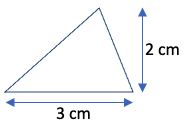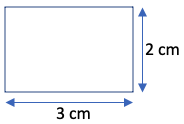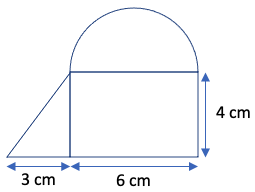Part 9: Geometry and Measurement

# 9.3: Area and perimeter

Notes

Many area and perimeter calculations in elementary math can be accomplished knowing just a few formulas based on circles, triangles, and rectangles.

• The area of a circle is given by π x r2, where r is the radius and π (pi) is the number 3.141592654… For hand calculation, we’ll simply use π = 3.14.
• The circumference of a circle is given by 2 x π x r.
• The area of a triangle is given by (1/2) x b x h, where b is the base and h is the height (perpendicular to the base). Any convenient side of the triangle can be selected as the base.
• We can use the Pythagorean theorem from 9.1: Angles to calculate one side length of a triangle from the other two side lengths.
• The area of a rectangle is given by l x w, where l is the length and w is the width.
##### Examples
1. Calculate the area of a circle with a radius of 5 cm. Area = π x r2 = π x 52 = π x 25 = 25π = 25(3.14) = 78.5 cm2.
2. Calculate the circumference of a circle with a radius of 5 cm. Circumference = 2 x π x r = 2 x π x 5 = 10π = 10(3.14) = 31.4 cm.
3. Calculate the area of the following triangle:Area = (1/2) x b x h = (1/2) x 3 x 2 = 3 cm2.
4. Calculate the area of the following rectangle:Area = l x w = 3 x 2 = 6 cm2.
5. Calculate the area of the following shape comprised of a half-circle, right triangle, and rectangle:Half-circle area = (1/2) x π x r2 = (1/2) x π x (6/2)2 = 4.5π = 4.5(3.14) = 14.13 cm2.
Triangle area = (1/2) x b x h = (1/2) x 3 x 4 = 6 cm2.
Rectangle area = l x w = 6 x 4 = 24 cm2.
Total area = 14.13 cm2 + 6 cm2 + 24 cm2 = 44.13 cm2.
6. Calculate the perimeter of the shape in Example 5:
Half-circle circumference = (1/2) x 2 x π x r = π x (6/2) = 3π = 3(3.14) = 9.42 cm.
Hypotenuse of right triangle = √(32 + 42) = √25 = 5 cm.
Perimeter = 9.42 cm + 5 cm + 3 cm + 6 cm + 4 cm = 27.42 cm.

The video below works through some area and perimeter calculations based on circles, triangles, and rectangles.

Practice Exercises

Do the following exercises to practice calculating areas and perimeters based on circles, triangles, and rectangles.